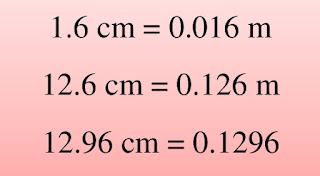# Conversion of Units of Length

Lesson Planning of Conversion of Units of Length (Meters into Centimetres)

Subject Mathematics

Students` Learning Outcomes

• Convert meters into centimetres and vice versa.

Information for Teachers

• A centimeter is a unit of length which is denoted as cm.
• Cent means 100 therefore, 1 m = 100 cm.
• Multiply the meters with 100 to convert them in centimetres, so, cm = m x 100.
• Divide the centimetres with 100 to get meters, so, as; m = cm ÷ 100
• Centimeter is used to measure small lengths.
• A centimeter is approximately the width of the fingernail of an adult person.
• Centipede is called by this name because it has 100 legs.
• During this lesson the teacher should consult with textbook at each level where required.

Material / Resources

Writing board, chalk/marker, duster, measuring tape, scale, textbook

Introduction

• Write ‘meter and centimeter’ on the board and ask the students to share, whatever they know about these units.
• Ask the students have you seen your scale, there are two measuring units on it i.e. inches and centimetres.
• Tell them that today we will learn how to convert meters into centimetres and centimetres into meters.

Development

 Activity 1 Tell the students that we use meters to measure relatively short lengths as compare to kilometres. Normally we use measuring tape and meter rod to measure such sort of lengths.Tell them that 1 m = 100 cm Take an example of teacher`s table and tell them about the short length conversions.Measure the length of the table and tell the students it is 2 meters long. Now we will convert the length of table into centimetres. To convert meters into centimetres simply multiply meters with 100, as; 1 m = 100 cm length of table = 2 cm cm = m x 100 length of table in cm = 2 m x 100 = 200 cm

 Activity 2 Conversion from centimetres to meters. Ask a student to measure length of classroom window in centimetres and write it on the writing board so it can be converted into meters. Suppose student tells you that length of window is 300 cm. Teach the students with the help of board. m = cm ÷ 100 length of window = 300 cm length of the window into meters,  3 meters = 300 ÷ meters Write the following questions and call various students to fill the blanks, as;Activity 3 Tell the students that now we will talk about the meters and centimetres of decimal number. Ask them, do they remember that they have adopted which method to correct the meters and kilometres into each other in the previous lesson? Encourage them on the correct answers. Tell them that in decimal numbers to correct the meters into centimetres the decimal point is moved to places to the right, as; 2.5 m = 250.0 or 250 cm. Tell them further that in decimal numbers to convert the centimetres into meters the decimal point is moved two places to the left, as;Sum up / Conclusion

• 1 m = 100 cm
• Multiply the meters with 100 to convert into centimetres, as; cm = m x 100
• To convert centimetres into meters we divide the centimetres with 100, as; m = cm ÷ 100.
• To convert the meters into centimetres in the decimal numbers move the decimal point to two places on the right.
• To convert the meters into centimetres in the decimal numbers move the decimal point to two places on the left.

Assessment

• Complete the following table.
• Give some questions to solve from the text book.